# What is counting sort?

Counting sort is a sorting algorithm, which can be used for sorting objects depending on the key values which are small in numbers. It stores the count of similar key values in some data structures like an array. The counting sort algorithm is primarily used when the range of the key values is not so big(difference between the minimum and maximum values), otherwise, it may lead to an increase in the space complexity.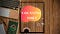COUNTING SORT

# Approach:

Working of counting sort depends on 4 steps:

## Range (value of k), Counting, Cumulative, Sorting :

Rang (value of k): From the given array find the minimum and maximum value of the array. For finding the min, max elements from an array, we need to scan the full array at least once which takes the time complexity of the order of n times ( i.e. O(n)).

Counting: Next, we have to count the number of occurrences of each key-value and store them in an array (lets count[] ) of the size range ( i.e. k). For counting the occurrence we have to travel the full array once and increment the corresponding key address in the array count[]. This process will take the time complexity of the order of n times ( i.e. O(n)).

Cumulative: As we know the counting sort is a stable sort, to maintain its stability, we need to perform compounding in the count array. Compounding is adding the previous element of the array to the next element. It takes the time complexity of the order of k times ( i.e. O(k)) where always k<=n.

Sorting: for sorting and maintaining the stability of the array, we will start the sorting from the end of the array. We will place each of the elements to its correct position in a new array using the compounded count array, and simultaneously decrease the respective value in the same. This process will take the time complexity of the order of n times ( i.e. O(n)).

# What is the Time complexity of counting sort?

As we saw in the above steps we have the total time complexity as:

Total time of f(n) = n + n + k + n = 3n+k

So, here we get big O of f(n) as O(n+k).

Worst case: O(n+k)

Best case: O(n+k)

Average case: O(n+k)

# Example and explanation?

Let us take an example of an array M[] with 17 elements in it.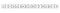Array M[] with 17 elements

Range: First to find out the range of the array we will find the maximum value and assume the lower bound of the range to be zero by default for the ease of code. So, the range of the array M is 0–9 that is 9.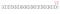The range is from 0 to 9, k=9

Counting: Now we will count the frequency of each key-value and store it in an array of size (range+1) i.e. 9+1=10.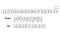Array Count of size 10

Cumulative: here we will add the previous element of the array to the current element and store the result in the same or different array (according to the requirement of the problem).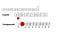Compounded array

Sorting: in the last step we will place all the elements in their right position and make a stable sort. For this, we will traverse the array from the end and will place the element in a new array at the position mentioned in the compounded array-1. Also, simultaneously we will reduce the value of frequency by 1 for the same key value in the compounded array.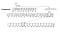Sorting in a stable way

And thereby we will get our sorted array in a stable form.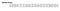Stable Sorted Array

# Application and approach?

• Counting sort can be used to sort an array with small differences between the min and max elements and have a higher number of occurrences.
• It can be used to count the frequency of the elements.
• Can be used to find the correct position of the element in case of multiple occurrences of elements (stable sort).

# Advantages of counting sort.

Linear Time Complexity: There are many sorting algorithms in DSA. Bubble, selection merge heap, etc. all these sorting algorithms are comparison-based sorts, where we compare the values, and based on the compression we may or may not swap the values. But if we talk about the counting sort, it is not a comparison-based sort, which does not lower bound it with O(nlogn).

# Disadvantages of counting sort.

• Restricted inputs: Counting sort is only effective/efficient when the range of potential elements in the array is small ( difference between max and min key values ) and the frequency of elements is high.
• Space cost: As the space complexity of count sort is n+k, the cost of extra k spaces is added to the total cost, leading to more space complexity. Hence it is not efficient for the big range of potential values.
• Can not be used with the negative elements in the array.

# How to Identify Counting sort problems?

If We have a small range key-value array with a high frequency of key values, then counting sort is best to use in this case.

Also, for selecting any sorting algorithm for any problem points we need to focus upon are the time complexity, space complexity, and the format expected for the given list (unstable or stable):

Time complexity: under the favorable condition of counting sort, it provides us the linear time complexity which is best of all sorting algorithms.

Space complexity: if space cost is not an issue to the user, then counting sort can be used as it takes the space complexity of n+k, which is higher than some of the other sorting algorithms.

Format of the array: it can successfully fulfill the requirement of a stable format as counting sort is a stable sort.

# Conclusion

Counting sort is one of the stable sort algorithms that can be relatively used for a smaller set of numbers with a small range and high frequency of key values.

Counting sort is not a comparison sort that removes the constraints of O(nlogn) time complexity, and it can give us a sorted array in linear time i.e. O(n+k) time (O(n+k)~=O(n) some times).